Definitions of Square Dance Calls and Concepts
Exchange The Triangle [C3A]

Index -->  Plus  |  A1  |  A2  |  C1  |  C2  |  C3A  |  C3B  |  C4  |  NOL  |
Definitions (Text Only) -->  Plus  |  A1  |  A2  |  C1  |  C2  |  C3A  |  C3B  |  C4  |  NOL  |
 Find call:

 Language: \$B8@8l(B Språk: Jazyk: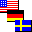All

From Twin Triangles. EN: 10
Twin Triangles (1 \$BBP\$N(B Triangle) \$B\$+\$i!%(B JP: 10
Från Twin Triangles. SE: 10
Z formace Twin Triangles. CZ: 10

Do a total of three Triangle Circulates except that when you become 1) the Apex dancer (for Apex-to-Apex Triangles), or 2) the In-Facing Base dancer (for Base-to-Base Triangles); do your next Circulate to the other Triangle. (From Apex-to-Apex Triangles, the Circulate is an Interlocked Triangle Circulate; from Base-to-Base Triangles, the Circulate is to the diagonal opposite position.) Exchange The Triangle can be fractionalized into thirds. EN: 20
\$B\$N(B Triangle \$B\$X(B Circulate \$B\$7\$^\$9!%(B (Apex-to-Apex Triangles \$B\$+\$i\$N(B Circulate \$B\$O(B Interlocked Triangle Circulate \$B\$H\$J\$j(B, Base-to-Base Triangles \$B\$+\$i\$N(B Circulate \$B\$OExchange The Triangle \$B\$O(B 3 \$B\$D\$N%Q!<%H\$KJ,\$1\$i\$l\$^\$9!%(B JP: 20
Gör totalt tre Triangle Circulate utom att när 1) du blir Apex dansare (i Apex-to-Apex Triangles), eller 2) du blir In-Facing Base dansare (i Base-to-Base Triangles); gör då din nästa Circulate till den andra Triangle. (Från Apex-to-Apex Triangles, är Circulate en Interlocked Triangle Circulate; från Base-to-Base Triangles, är det Circulate till den diagonalt motsatta positionen.) Exchange The Triangle kan fraktionaliseras i tredjedelar. SE: 20
Jděte 3x Triangle Circulates, ale když se stanete buď 1) tanečníkem na vrcholu (Apex) (ve formaci Apex-to-Apex Triangles), nebo 2) In-Facing tanenčík na základně (ve formaci Base-to-Base Triangles); jděte svůj Circulate do druhého Triangle. (Z formace Apex-to-Apex Triangles je Circulate jako Interlocked Triangle Circulate; z formace Base-to-Base Triangles je Circulate diagonálně do protilehlé pozice.) Figura Exchange The Triangle může být rozdělena na třetiny. CZ: 20

From Apex-to-Apex Wave-Based Triangles (Very Centers lead) EN: 30
Apex-to-Apex Wave-Based Triangles \$B\$+\$i(B (Very Centers \$B\$,%j!<%I(B)JP: 30
Från Apex-to-Apex Wave-Based Triangles (Very Centers leder) SE: 30
Z formace Apex-to-Apex Wave-Based Triangles (Very Centers vedou) CZ: 30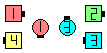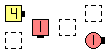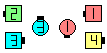before före před Exchange The Triangles\$B\$NA0(B the left Triangle after 1/3... EN: 40 \$B:8\$N(B Triangle 1/3 \$B\$N8e(B... JP: 40 vänster Triangle efter 1/3... SE: 40 levý Triangle po 1/3... CZ: 40 after efter po \$B8e(B
From Base-to-Base Tandem-Based Triangles (Trailing Centers lead) EN: 50
Base-to-Base Tandem-Based Triangles \$B\$+\$i(B (Trailing Centers \$B\$,%j!<%I(B)JP: 50
Från Base-to-Base Tandem-Based Triangles (Trailing Centers leder) SE: 50
Z formace Base-to-Base Tandem-Based Triangles (Trailing Centers vedou) CZ: 50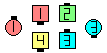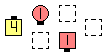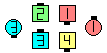before före před Exchange The Triangles\$B\$NA0(B the left Triangle after 1/3... EN: 60 \$B:8\$N(B Triangle 1/3 \$B\$N8e(B... JP: 60 vänster Triangle efter 1/3... SE: 60 levý Triangle po 1/3... CZ: 60 after efter po \$B8e(B

Note: As with all Exchanges, do Circulates staying on the inside of your formation until the crossover point, and then do Circulates staying on the outside of the other formation (and adjust to end on footprints of the original formation). EN: 70
\$BCm\$N(B formation \$B\$N30B&\$r(B Circulate \$B\$7\$^\$9!%\$=\$7\$F%*%j%8%J%k\$N(B formation \$B\$N(B footprint (\$BLuJP: 70
Notera: Som vid alla Exchange, gör Circulates och stanna på insidan av din formation tills du kommer till den punkt där du korsar över och gör sedan Circulates och stanna på utsidan av den andra formationen (och justera för att sluta på 'footprints' på den ursprungliga formationen). SE: 70
Poznámka: Stejně jako u všech Exchange, jděte Circulates uvnitř své formace až do místa přechodu do druhé formace, pak jděte Circulates vnějškem (a srovnejte se do pozic původní formace). CZ: 70

Exchange The 3 By 1 Triangle [NOL]
(Very Centers \$B\$,%j!<%I(B) JP: 80
(Very Centers leder): SE: 80
(Very Centers vedou): CZ: 80
(do a total of 4 Circulates). EN: 85
(Ciculate \$B\$r9g7W(B 4 \$B2s9T\$\$\$^\$9(B). JP: 85
(gör totalt 4 Circulate). SE: 85
(jděte celkem 4x Circulate). CZ: 85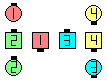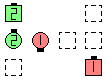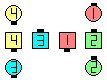before före před Exchange The 3 By 1 Triangles\$B\$NA0(B the left Triangle after 1/4... EN: 90 \$B:8\$N(B Triangle 1/4 \$B\$N8e(B... JP: 90 vänster Triangle efter 1/4... SE: 90 levý Triangle po 1/4... CZ: 90 after efter po \$B8e(B
Exchange The 3 By 1 Triangles [NOL]
(Very Centers \$B\$,%j!<%I(B) JP: 100
(Very Centers leder): SE: 100
(Very Centers vedou): CZ: 100
(do a total of 4 Circulates). EN: 105
(Ciculate \$B\$r9g7W(B 4 \$B2s9T\$\$\$^\$9(B). JP: 105
(gör totalt 4 Circulate). SE: 105
(jděte celkem 4x Circulate). CZ: 105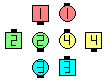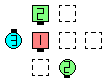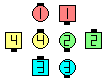before före před Exchange The 3 By 1 Triangles\$B\$NA0(B the left Triangle after 1/4... EN: 110 \$B:8\$N(B Triangle 1/4 \$B\$N8e(B... JP: 110 vänster Triangle efter 1/4... SE: 110 levý Triangle po 1/4... CZ: 110 after efter po \$B8e(BCALLERLAB definition for Exchange The TriangleChoreography for Exchange The TriangleComments? Questions? Suggestions?Page translated by David Tesař.